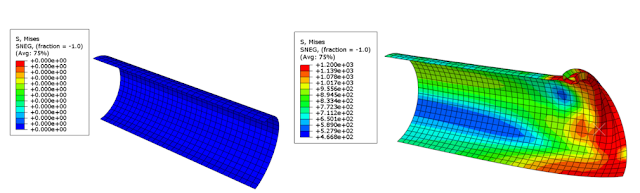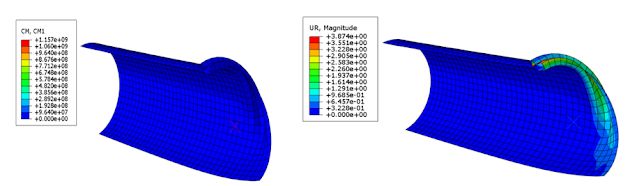## Welcome to the World of Modelling and Simulation

### What is Modelling?

This blog is all about system dynamics modelling and simulation applied in the engineering field, especially mechanical, electrical, and ...### Simulation of Buckling Behavior of a Pipe by Abaqus

In this tutorial, I am going to show how we can simulate the buckling characteristics of a pipe in Abaqus. The length of the pipe is 4.5 m, outside diameter (OD) is 0.9 m, and thickness is 9 mm. First, let us consider the stress-strain relationship, which is defined by the following table.

Table 1: Stress-Strain Relation for a Pipe

True Strain     True Stress (N/mm^2)
0                              0
0.001                     200
0.002                     400
0.003                     600
0.004                     800
0.0055                     900
0.008                     1000
0.0125                     1100
0.026                     1200

The relationship between the true and engineering stress-strain is governed by:

σtrue = F/A = (F/A0) (1+εeng) = σeng(1+εeng)

Now, to find the elastic strain, the following relation is used.

εe = σ/E

The following table is based on the above definitions.

Table 2: Elastic and Plastic Strain for a Pipe

 TrueStrain True Stress (N/mm^2) Tr Stress Elastic Strain (εe) Plastic Strain (εp) 0 0 0 0 0 0.001 200 200.2 0.001001 -1e-06 0.002 400 400.8 0.002004 -4e-06 0.003 600 601.8 0.003009 -9e-06 0.004 800 803.2 0.004016 -1.6e-05 0.0055 900 904.95 0.004525 0.000975 0.008 1000 1008 0.00504 0.00296 0.0125 1100 1113.75 0.005569 0.006931 0.026 1200 1231.2 0.006156 0.019844

The internal pressure inside the pipe is given by following relation:

P = [0.8(2t)σy]/OD

P = 12.8 MPa

This is the ultimate internal pressure of the pipe. Now, we are ready to create the model in Abaqus.

Steps in Abaqus Modelling:

Creating the Geometry
First, a part is created in Abaqus CAE, where a 3-point arc is chosen to draw a half circle with radius 450 mm. Since, the part is selected as 3D shell with extrusion, the length is 2250 mm. We will use symmetry and that’s why, these dimensions are selected to use symmetry boundary conditions about X and Z-axes.

The S4R shell element is chosen with reduced integration for analysis.

Meshing

Next, we create the mesh. The mesh size is set to 18 mm, which is twice the size of the shell thickness.

Creating Section
After that, the sections are created. The shell thickness is set 9 mm as per the problem.

Assigning Section
After creating the section, we assign it to the part. Then, we use “Render Shell Thickness” to show the shell thickness.

Creating Partition
A partition is created with 200 mm offset from one of the pipe edges.

Setting Material Properties

For the elastoplastic behaviour, the following material properties are given:Creating Steps with Loads and Boundary Conditions

In this step, we define the boundary condition (BC) and load. A reference point (RP) is created to fix one end of the pipe. For the RP, a rigid body constraint is defined. Three BCs are generated as follow:

BC-3: for the reference point (RP1), which is fixed along the axes.

Then, a pressure load of magnitude 12.8 MPa is applied inside the pipe.

Results and Discussions:

Since the model is created with necessary boundary conditions and loads, in this section, we are interested to consider two circumstances.

Buckling when both pressure and bending are considered

Buckling when bending is considered without pressure

We apply the static Rik’s approach as a force control. The following figure shows the Von Mises stress when only pressure is applied inside the wall of the pipe.

Buckling when both pressure and bending are considered

At first, we apply a moment of 5x10^10 Nmm at the reference point by creating a new step where Rik’s static algorithm is selected. In this scenario, the pressure is still present inside the pipe. The following figure demonstrates the three steps for the simulation.The following figure shows two relationship when pressure is present: moment vs arc length and rotation vs arc length. The intersection point between two graphs in the figure is the convergence point for the simulation.Showing relationships between moment and arc length, and rotation and arc length with pressureThe following plot shows the relation between moment and angular displacement when pressure is present in the pipe.Buckling when bending is considered without pressure

In this step, we just suppress the load leaving all other conditions same. We are interested to see the effect of the bending only on the buckling rate of the pipe.Showing 2 steps: no pressure (left), and with angular displacement (right)The following figure shows two relationship when pressure is absent: moment vs arc length and rotation vs arc length. The intersection point between two graphs in the figure is the convergence point for the simulation.Showing relationships between moment and arc length, and rotation and arc length without pressureMoment without pressure (left) and rotation without pressure (right) when arc length is 0.8016 mm

The following plot shows the relation between moment and angular displacement when pressure is absent in the pipe.From the following comparison, we see that the buckling is higher when there is no pressure than the buckling with internal pressure.To learn in detail of FEA, you may visit here.

#VonMisesstress #Buckling #Bending #FiniteElementAnalysis #FEA #Abaqus #ModellingSimulation #Blog #Blogger

1.nice info!! thanks
2.3.